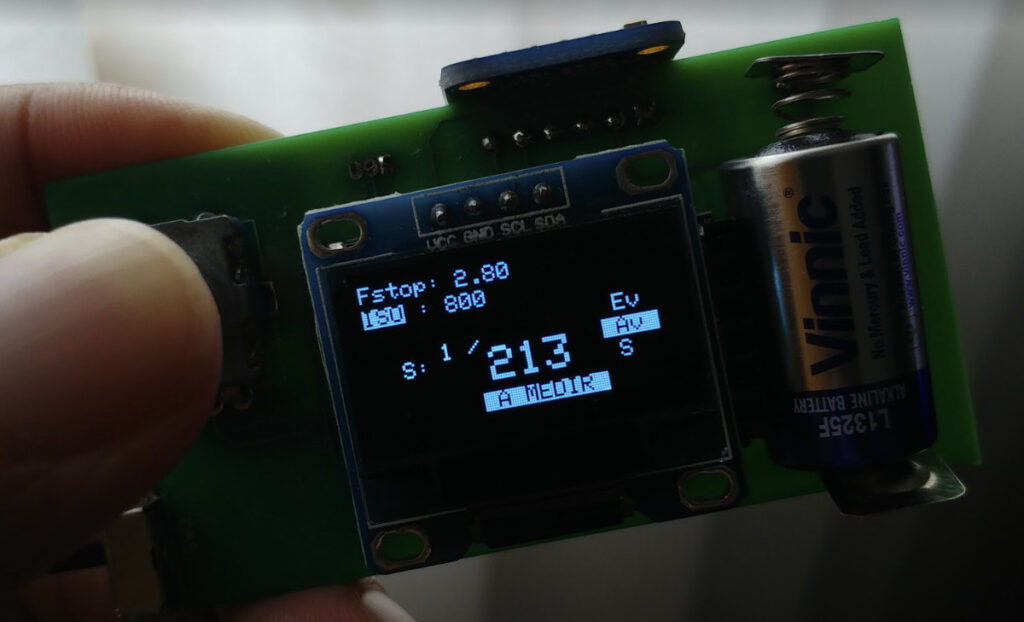# Measure Light – The quest for a light meter for analogue photography – Part One

I love analogue photography, even more when it’s with fully mechanical medium format cameras, such as the Flexaret Automat VII, and the Hasselblad 500c.

However, where are some situations where the correct exposure is difficult to determine. On such cases, one could use a second camera for TTL metering (such as DSLR), a phone app…or a light meter.

On my location, an new sektronick light meter price varies from 109 € to 600 € depending on the model. On ebay you could get an used, cheaper , vintage light meter, but your mileage might vary.

So since the price and availability of light meters were not to my liking, I’ve decided to build my own from scratch, based on an arduino board, with some help of Pedro Virtebo, at Maquinas de Outros Tempos.

Bear in mind this is not the first or last time anyone has done something similar, a simple google search show a ton of similar projects, with different levels of polish. But just implementing these would not give me enough understanding of how a light meter works.

## Main Goals

The main goals for this project were :

• Have a finished, usable light meter.
• Have the output in Ev, in order to use the Ev scale on my medium format cameras.
• Understand the relationship between Lux, Ev, Fstop and Aperture.
• No user settings – usage must be as simple as possible.

## Hardware

### Hardware baseline

• Arduino Based
• TSL 2591 Light sensor
• Single encoder for user interface

### Hardware Architecture

The light meter consists of an Adafuit TSL2591 and a OLED display connected via i2c bus, with an encoder for user interaction.

The arduino board chosen was the Arduino mini pro, due to its size.

In regards to the schematic, there are not many ways to connect I2C peripherals (the Light sensor and the OLED display) to an Arduino mini pro.

In fact, one could use f_lux code, with some minor changes, to work with this PCB.

## Software

### Getting the numbers

The TSL2591 only outputs the Lux value at measurement time.
In order to have values that are directly usable for photography there are some calculations to be performed.

As a start, its simpler to determine the Exposure Value from the Lux measurement (instead of trying to get fstop or shutter speed first) :

Ev_{100}  = Log_2(\frac{Lux}{2.5} )

However, the Arduino libraries do not have a Log2(x), but a Log10(x) instead. Dusting off the math in order to use Log10(x) instead :

Ev_{100}  = \frac {Log_{10}(\frac{Lux}{2.5} )}{Log_{10}(2)}

However, this is only valid for ISO 100.
In order to have the Ev compensated for an arbitrary ISO value, the formula becomes :

Ev_{iso} = \frac {Log_{10}(\frac{Lux}{2.5} )}{Log_{10}(2)} + \frac{Log_{10}(\frac{iso}{100})}{Log_{10}(2)}

Now that we have the Ev, at this stage the light meter is already usable on cameras that have an Ev scale.

The Ev result is the basis for the Fstop or shutter speed calculation.

#### Fstop calculation

Now that we have an Ev value we can now calculate the correct Fstop for a given shutter speed :

fstop = \sqrt {2^{Ev} * shutter\_speed}

#### Shutter Speed calculation

shutter\_speed = \frac {Fstop^2}{2^{ev}}

Obviously, we want a fraction as a result (1/x) , not a floating point number (like 1/2 instead of 0.5) , thus :

denominator = \frac{1.0}{Shutter\_speed}

Of course, the disadvantage is the fact that the output of these functions might not fall into the pre-detemined Shutter speed or Fstop values selectable on the camera (1, 1/2, 1/1000, or f1.8, f4, f5.6 for example). In the future I might add some form of rounding to the nearest selectable value on the light meter code.Using the formula we get a “correct” result, but its one not directly selectable on a camera. In this case it should be rounded to 1/200 of a sec.

End of part one.